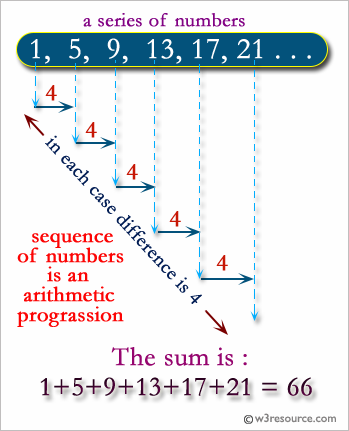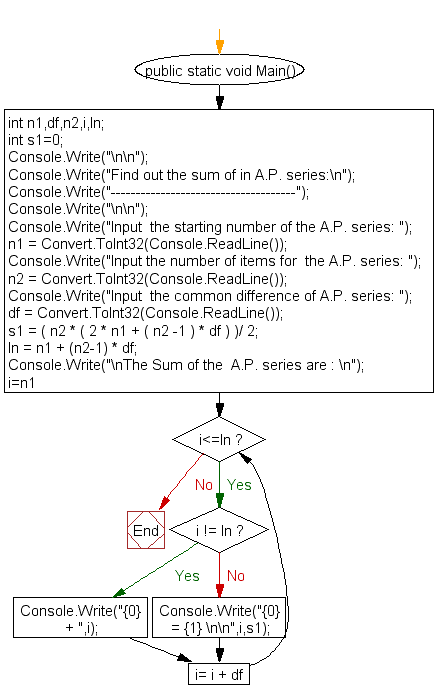﻿ C# - Find out the sum of in A.P. series - w3resource# C# Sharp Exercises: Find out the sum of in A.P. series

## C# Sharp For Loop: Exercise-49 with Solution

Write a C# Sharp program to find out the sum of in A.P. series.Sample Solution:-

C# Sharp Code:

``````using System;
public class Exercise49
{
public static void Main()
{

int n1,df,n2,i,ln;
int s1=0;

Console.Write("\n\n");
Console.Write("Find out the sum of in A.P. series:\n");
Console.Write("-------------------------------------");
Console.Write("\n\n");

Console.Write("Input  the starting number of the A.P. series: ");

Console.Write("Input the number of items for  the A.P. series: ");

Console.Write("Input  the common difference of A.P. series: ");

s1 = ( n2 * ( 2 * n1 + ( n2 -1 ) * df ) )/ 2;
ln = n1 + (n2-1) * df;
Console.Write("\nThe Sum of the  A.P. series are : \n");

for(i=n1;i<=ln; i= i + df ){
if (i != ln)
Console.Write("{0} + ",i);
else
Console.Write("{0} = {1} \n\n",i,s1);
}
}
}
```
```

Sample Output:

```Find out the sum of in A.P. series:
-------------------------------------
Input  the starting number of the A.P. series: 0
Input the number of items for  the A.P. series: 10
Input  the common difference of A.P. series: 5
The Sum of the  A.P. series are :
0 + 5 + 10 + 15 + 20 + 25 + 30 + 35 + 40 + 45 = 225
```

Flowchart:C# Sharp Code Editor:

Contribute your code and comments through Disqus.

What is the difficulty level of this exercise?

Test your Programming skills with w3resource's quiz.

﻿